# Parallelogram Problems

#### Number of problems found: 38

• ParallogramParallogram base is 24 cm and high 10 cm. How many such tile are required to cover a floor of area 1080m2?
• A perimeter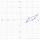A perimeter of a parallelogram is 2.8 meters. The length of one of its sides is equal to one-seventh of the entire perimeter. Find lengths of the sides of the parallelogram.
• What is 10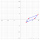What is this The area of a parallelogram that has vertices with the coordinates (0, 0), (4, 0),(5, 3), and (1, 3)?
• Parallelogram diagonalsFind the area of a parallelogram if the diagonals u1 = 15 cm, u2 = 12 cm and the angle formed by them is 30 degrees.
• Parallelogram ABCDWe have the parallelogram ABCD, where AB is 6.2 cm BC is 5.4 cm AC is 4.8 cm calculate the height on the AB side and the angle DAB
• Tangens parallelogramIf ∠BAD between the sides AB and AD of the parallelogram is θ, what is tan θ? See diagram: A=(7,1) B=(5,-2) C=(12,1) D=(14,4)
• The parallelogram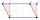The parallelogram has one side 2 dm long, which is one-sixth of its circumference. How many cm does the other side of the parallelogram measure?
• ParallelogramFind the parallelogram's perimeter, where base a = 8 cm, height v = 3 cm, and angle alpha = 35° is the magnitude of the angle at vertex A.
• CoordinatesDetermine the coordinates of the vertices and the content of the parallelogram, the two sides of which lie on the lines 8x + 3y + 1 = 0, 2x + y-1 = 0 and the diagonal on the line 3x + 2y + 3 = 0
• Diamond area from diagonalsIn the diamond ABCD is AB = 4 dm and the length of the diagonal is 6.4 dm long. What is the area of the diamond?
• Garden exchangeThe garden has a rectangular trapezoid shape, the bases of which have dimensions of 60 m and 30 m and a vertical arm of 40 m. The owner exchanged this garden for a parallelogram, which is 7/9 of the area of a trapezoidal garden. What is the size of the ne
• Parallerogram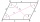In parallelogram ABCD is a = 2.5 cm and height to side a is 7.2 cm and height to side b is 4 cm. Find the side length b.
• Parallelogram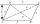The sides of the parallelogram are 8 cm and 6 cm long and the angle of the diagonals is 60°. What is its area?
• Children playgroundThe playground has a trapezoid shape, and the parallel sides have a length of 36 m and 21 m. The remaining two sides are 14 m long and 16 m long. Find the size of the inner trapezoid angles.
• Parallelogram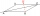Rhomboid (parallelogram) has a longer side of 50 cm long. The size of its one height is four times the size of its second height. Calculate the length of the shorter side of this rhomboid in the centimeters.
• Parallelogram perimeter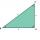The ABC triangle with sides a = 5cm, b = 3cm, c = 40mm has the center of the sides of the K,L,M. How many cm have the KBLM parallelogram perimeter?
• Parallelogram +ľ| AB | = 76cm, | BC | = 44cm, angle BAD = 30 °. Find the area of the parallelogram.Show that the quadrilateral with vertices P1(0,1), P2(4,2) P3(3,6) P4(-5,4) has two right triangles.Side a of parallelogram is twice longer than side b. Its circumference is 78 dm. Calculate the length of the sides of a parallelogram.Calculate the missing side of a parallelogram, if you know the perimeter o and one side: a) o = 7.2 cm; b = 1.8 cm b) o = 5.4 cm; a = 1.9 cm